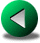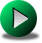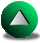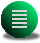Previous: Results
Up: Single Gyre Circulation in

# Scale Analysis and Discussion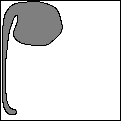In order to interpret the large scale and steady characteristics of the Munk circulation of the previous sections, we propose to develop some scaling arguments and compare these with the numerical results. One approach is to derive a criterion based on vorticity budgets (following Pedlosky) under the free-slip boundary condition for steady solutions which allows for curved boundaries. For straight walls, the relative vorticity is zero along the boundary. For a strong inertial layer in which we neglect viscous effects, we know that the absolute vorticity is conserved along a parcel trajectory (see Figure 5.18). Let us consider the conservation of absolute vorticity for simplicity. This one is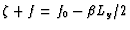in the southern part of the domain (upstream of the inertial layer) where the relative vorticity is zero. Since absolute vorticity is conserved in the inertial layer, it is reasonable to imagine that the minimum absolute vorticity is such that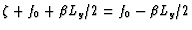in the northern part of the domain (downstream of the inertial layer). Therefore, the minimum relative vorticity is. Hence, in order to have an idea of the magnitude of the viscous flux of vorticity out of the domain, we only need to estimate the width of the viscous sublayer in the presence of the recirculation. One limitation, though, of this approximation is that the recirculation is formed of closed contours of both potential vorticity and the streamfunction. Hence, to the extent that particles remain trapped in the recirculation for long periods, wind forcing can lead to even lower values ofhere. However, from experience, the minimum in the recirculation zone is usually superior to (less negative) or about the number we gave (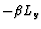), as long as the recirculation zone is confined to the northwestern part of the gyre. As soon as this zone reaches the eastern walls (i.e., with increased Re), it forms a Fofonoff-type gyre that fills the whole basin, and for which the vorticity is much lower. Using the vorticity budget given in (5.1) and the viscous sublayer thickness,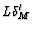, as the scale at which the vorticity varies in the boundary layer, we derive the simple criterion for a circulation in straight walls in the presence of a strong recirculation (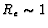):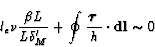(5.17)

where le is the length of the recirculation. The second term, the wind input, in the vorticity balance is easy to determine. It varies with h; however, from experience, we can consider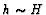. A first scaling for le can be obtained from (5.17) after substituting (5.2-5.5):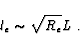(5.18)

For Re=0.5, we obtain le=710 km, which is reasonably close to what is observed in Figure 5.5. This scaling can be compared to Pedlosky's (1996, pages 85-86). Pedlosky evaluates the recirculation length scale using three equalities. The first one comes from the equality in the momentum equations between the advection terms and the diffusion terms in the viscous sublayer in the presence of the recirculation: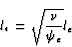(5.19)

where l* is the thickness of the sublayer and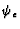is the transport in the recirculation. The second equality comes from the transport in the sublayer being equal to the Sverdrup transport and from the velocity in the sublayer being equivalent to the velocity at the edge of the recirculation: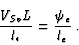(5.20)

The third equality comes from the vorticity input by the wind in the interior of the ocean being equal to the viscous flux of vorticity across the recirculation edge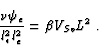(5.21)

These three combined equations allow one to derive one equation for le: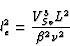(5.22)

which can be simplified using (5.2-5.5) to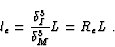(5.23)

Pedlosky's scaling of le however implies an inconsistent definition of the viscous boundary layer thickness along the recirculation. The thickness for the viscous sublayer can be obtained from (5.19-5.23):(5.24)

Using again (5.2-5.5), this equation becomes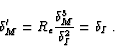(5.25)

Hence, the viscous sublayer thickness is no longer dependent on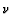. This seems counter-intuitive: for high Re, one expects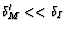. Therefore, we will continue to use our own estimate ofas given in (5.5) and we will give the equivalent result usingin the presence of a recirculation as given by Pedlosky. The true scaling might be in between these two values. Nonetheless, in both cases, the behavior of le with increasing Reynolds number is roughly the same. The recirculation length quickly increases with increasing Reynolds number and the Sverdrup interior can no longer be sustained for Re> 1.

Now, in the presence of a curved coastline, (5.17) is modified to account for the positive vorticity produced at the wall. Since the recirculation has closed contours of streamlines, all the Sverdrup transport goes between the recirculation and the wall. We assume this region to be the viscous sublayer. Therefore, the volume transport through the sublayer is:(5.26)

where v* is the velocity in the viscous sublayer (this is actually identical to Eq. 5.20)). This yields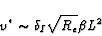(5.27)

for the scaling of v*. Using (5.26), we estimated v* to be of the order of 0.84 m/s for Re=3.5. We now need to estimate the vorticity produced at the wall. This is of the order of v*/Rs as given by (5.7). Let us assume that the production of positive vorticity is valid within half a wavelength of the curvy coastline and that no vorticity is produced in the other half. The second term in (5.1) becomes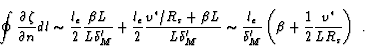(5.28)

Therefore, in the presence of curved coastlines, the relation (5.17) becomes: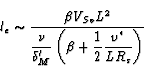(5.29)

Using the same values as above,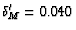at Re=0.5 and Rs=10 km, this relation yields a length of le=340 km.

There are two regimes, depending on the magnitude of Rs, the radius of curvature of the coastline; one at low curvature corresponding to (5.18) and the second at high curvature that we want to investigate. Let us consider now the condition under which the recirculation is controlled by the curvature of the coastline. This condition corresponds to the second term being larger than the first term in the denominator of (5.29):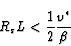(5.30)

and after substituting (5.5,5.26), we obtain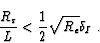(5.31)

Hence, the transition depends on the Reynolds number. As Re increases, the radius of curvature can increase for the second regime to persist, implying less curvy coastlines. For the second regime, the length of the recirculation is governed by the vorticity produced at the wall and, substituting (5.5,5.26), is scaled as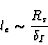(5.32)

Hence, the length of the recirculation in curved geometries is no longer dependent on the eddy-viscosity,. In other words, the Reynolds number dependence in le disappears. This result is valid as long as the curvature satisfies (5.31).

The weakest point in this argument is probably the estimate of the thickness of the viscous sublayer () and the velocity in the sublayer (v*) in the presence of the recirculation. Using the estimation of Pedlosky,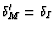, we would have found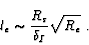(5.33)

By this estimation, le is still dependent on the Reynolds number, but the dependence would be somewhat weaker when compared to regular domains (Eq 5.18 or 5.23). Moreover, in the presence of curved coastline, it is not clear what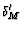becomes. The viscous sublayer might be squeezed between the recirculation and the bumps and therefore, the normal derivative of the vorticity might be increased, which favors smaller recirculations. It is also possible that the viscous sublayer is not squeezed but that some streamlines of the recirculation may lie within it. In such a case, a proper definition of the viscous sublayer becomes difficult. The second point relates to the form that the vorticity takes around the bumps, which we assume to be constant over a half wavelength of the curvy coastline. As we noted in the numerical results, the vorticity actually behaves quite singularly at the tip of bumps. An additional problem relates to the length of the perimeter which increases with increasing number of bumps and with increasing amplitude of these bumps. This would again favor lower values for le. Lastly, these scaling arguments were based on the assumption that the action of the transient eddies are negligible, which may not be the case for sufficiently high Re.

We now compare the predictions from our scaling argument about the recirculation length scale to Figure 5.5. We note that, at Re=0.5, the recirculation changes in character between Geometry II and III. Between these two geometries, the strength of the recirculation weakens and its length decreases. Moreover, for Geometry V, the length of the recirculation does not increase significantly with increasing Re (300 km to 350 km). The latter observation is consistent with (5.32). Therefore, the circulation seems to be controlled by the curvature of the geometry between Geometry II and III. At Re=0.5, the inequality (5.31) is however only satisfied for Geometry V. Moreover, for a fixed radius of curvature, (5.31) should be satisfied at a specific Re and the circulation in that particular geometry should be controlled by the radius of curvature beyond that specific Re. However, we observe the opposite. For example, as Re increases, the length of the recirculations for Geometry IV increases slowly but then jumps to the energetically high branch. The criterion (5.31) may not be very representative when the solution tends to jump to the energetically high branch with increasing Re. Nonetheless, the formula derived for le seems to yield a relatively good prediction when the curvature is large enough (Geometry V).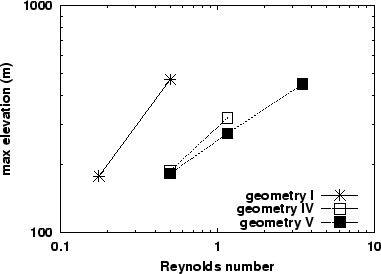Now focusing on results from Geometries I, IV and V, we derive a predictive law for the strength of the recirculation based on previous scalings. The Geometries IV and V are chosen because they show the most robust sign that their circulation is controlled by the curvature. The strength of the gyre is given by the maximum transport through the basin (dominated by the recirculation). Let us define the strength of the recirculation as the volume transport through it. The transport is defined as the mean velocity in the recirculation multiplied by its cross-section length. Let us assume that the magnitudes of v* and le are sufficient to characterize this transport. From (5.18) and (5.27), the maximum transport in regular basins is then given by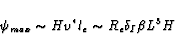(5.34)

and in irregular basins by, substituting (5.27) and (5.32)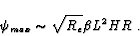(5.35)

Therefore the strength should be sensitive to the presence of the bumps. A good proxy for the strength of the gyre is the maximum elevation observed in the basin (because of the geostrophic approximation prevailing in most of the domain). Figure 5.19 shows the maximum elevation with respect to Re. It appears that the strength of the gyre is dependent on the form of the geometry. There is a clear shift in the strength of the gyre between Geometries I and IV. Moreover, the slope (the power relation between hmand Re or the slope coefficient in a log-log plot) is close to unity for the regular geometry (1.06), whereas it is about 0.5 for Geometry IV. At Re=0.5, the strength is identical for Geometry IV and V, but the slope is somewhat less for Geometry V. These results seem to follow (5.34) and (5.35), and the scaling for the sublayer thickness in the presence of the recirculation seems therefore to be closer to (5.5) than to that of Pedlosky.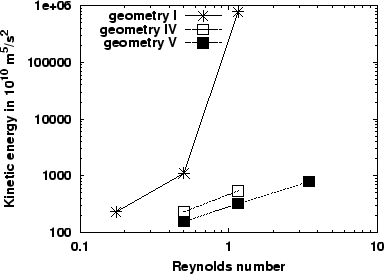Another important quantity related to the strength of the gyre is the kinetic energy (KE). We plot KE with respect to Re. Since the strength of the recirculation is dependent on the Reynolds number via v*, the kinetic energy must depend on the Reynolds number despite the presence of the indentations, but at much lower rate compared to the kinetic energy in regular geometries. By assuming that, at first order, KE is governed by the energy in the recirculation, we estimate that in the regular geometry the energy grows with both the length and the strength of the recirculation by using (5.18) and (5.27)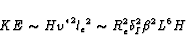(5.36)

whereas it grows only with the strength of the recirculation in the presence of indentations, substituting (5.27) and (5.32)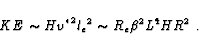(5.37)

Figure 5.20 shows the increase in the energy of the mean fields for Geometries I, IV and V. The presence of a wavy perturbation of the circular geometry retards the jump of the solution to the high energy branch and the increase with the Reynolds number is much slower in the presence of indentations along the coastline. Before the solution in the intermediate geometry jumps to the high branch, it is noteworthy that the slope for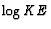with respect to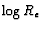, for the two irregular geometries, is rather similar. The slope is 1.7 for Geometry I, and 0.85 for Geometry IV and 1.0 for V. All actual values for the slopes are rather close to their expected values, even though this might be coincidental.Previous: Results